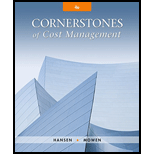# Algers Company produces dry fertilizer. At the beginning of the year, Algers had the following standard cost sheet: Algers computes its overhead rates using practical volume, which is 54,000 units. The actual results for the year are as follows: a. Units produced: 53,000 b. Direct materials purchased: 274,000 pounds at $2.50 per pound c. Direct materials used: 270,300 pounds d. Direct labor: 40,100 hours at$17.95 per hour e. Fixed overhead: $161,700 f. Variable overhead:$122,000 Required: 1. Compute price and usage variances for direct materials. 2. Compute the direct labor rate and labor efficiency variances. 3. Compute the fixed overhead spending and volume variances. Interpret the volume variance. 4. Compute the variable overhead spending and efficiency variances. 5. Prepare journal entries for the following: a. The purchase of direct materials b. The issuance of direct materials to production (Work in Process) c. The addition of direct labor to Work in Process d. The addition of overhead to Work in Process e. The incurrence of actual overhead costs f. Closing out of variances to Cost of Goods Sold### Cornerstones of Cost Management (C...

4th Edition
Don R. Hansen + 1 other
Publisher: Cengage Learning
ISBN: 9781305970663

#### Solutions

Chapter
Section### Cornerstones of Cost Management (C...

4th Edition
Don R. Hansen + 1 other
Publisher: Cengage Learning
ISBN: 9781305970663
Chapter 9, Problem 30P
Textbook Problem
83 views

## Algers Company produces dry fertilizer. At the beginning of the year, Algers had the following standard cost sheet:Algers computes its overhead rates using practical volume, which is 54,000 units. The actual results for the year are as follows: a. Units produced: 53,000 b. Direct materials purchased: 274,000 pounds at $2.50 per pound c. Direct materials used: 270,300 pounds d. Direct labor: 40,100 hours at$17.95 per hour e. Fixed overhead: $161,700 f. Variable overhead:$122,000 Required: 1. Compute price and usage variances for direct materials. 2. Compute the direct labor rate and labor efficiency variances. 3. Compute the fixed overhead spending and volume variances. Interpret the volume variance. 4. Compute the variable overhead spending and efficiency variances. 5. Prepare journal entries for the following: a. The purchase of direct materials b. The issuance of direct materials to production (Work in Process) c. The addition of direct labor to Work in Process d. The addition of overhead to Work in Process e. The incurrence of actual overhead costs f. Closing out of variances to Cost of Goods Sold

1.

To determine

Compute the direct materials price variance and the direct materials usage variance.

### Explanation of Solution

Direct material price variance: The variation in between actual price and estimated price paid for materials multiplied by the actual quantity is called material price variance. It is used to determine difference in price paid for material the price that was supposed to be paid for material.

The following formula is used to calculate direct material price variance:

Direct materials price variance=[(ActualPriceStandard Price)×Actual Quantity]

Direct material usage (efficiency) variance: It is a measure that determines the variation in between actual and standard quantity of input multiplied by the standard unit price is called material usage variance.

The following formula is used to calculate direct material usage variance:

Direct materials usage variance=[(ActualQuantityStandard Quantity)×Standard Price]

Compute the direct materials price variance:

Direct materials price variance=[(ActualPriceStandard Price)×Actual Quantity]=[($2.50 per pound$2

2.

To determine

Calculate the direct labor rate variance and labor efficiency variance.

3.

To determine

Calculate the fixed overhead spending and volume variance.

4.

To determine

Calculate the variable overhead spending and efficiency variances.

5.

To determine

Prepare journal entries for the given.

### Still sussing out bartleby?

Check out a sample textbook solution.

See a sample solution

#### The Solution to Your Study Problems

Bartleby provides explanations to thousands of textbook problems written by our experts, many with advanced degrees!

Get Started

Find more solutions based on key concepts
INTEREST RATE SENSITIVITY .An investor purchased the following 5 bonds. Each bond had a par value of 1,000 and ...

Fundamentals of Financial Management, Concise Edition (with Thomson ONE - Business School Edition, 1 term (6 months) Printed Access Card) (MindTap Course List)

Explain interest.

Intermediate Accounting: Reporting And Analysis

What is the difference between equity capital and debt capital?

Foundations of Business (MindTap Course List)